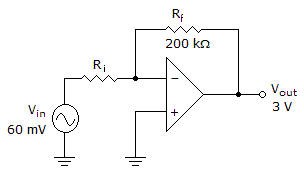# Electronics - Operational Amplifiers

### Exercise :: Operational Amplifiers - General Questions

36.

If an op-amp has one input grounded and the other input has a signal feed to it, then it is operating as what?

 A. Common-mode B. Single-ended C. Double-ended D. Noninverting mode

Explanation:

No answer description available for this question. Let us discuss.

37.

If the feedback/input resistor ratio of a feedback amplifier is 4.6 with 1.7 V applied to the noninverting input, what is the output voltage value?

 A. 7.82 V B. saturation C. cutoff D. 9.52 V

Explanation:

No answer description available for this question. Let us discuss.

38.

The Schmitt trigger is a two-state device that is used for:

 A. pulse shaping B. peak detection C. input noise rejection D. filtering

Explanation:

No answer description available for this question. Let us discuss.

39.

When a capacitor is used in place of a resistor in an op-amp network, its placement determines:

 A. open- or closed-loop gain B. integration or differentiation C. saturation or cutoff D. addition or subtraction

Explanation:

No answer description available for this question. Let us discuss.

40.What value of input resistance is needed in the given circuit to produce the given output voltage?

 A. 50B. 4 kC. 4.08 kD. 5 k